Previous: 2.3.4 Consistency with Bulk Up: 2.3 Closure Relations for Next: 3. Highest Order Moment

## 2.3.5 Hierarchy of Equations

Using the mobility closure given by Equation 2.57 the fluxes can be eliminated. The system of first order moment equations then becomes a system of second order equations in the even moments.

With the relaxation time approximation the general equation for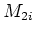is of the following form:(2.73)

Using the explicit expression for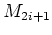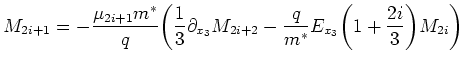(2.74)

we see thatenters Equation 2.74 only through the term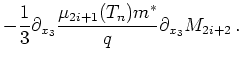(2.75)

This is the only second order term in Equation 2.74 and can be interpreted as a diffusion operator. The general equation for theequation can be supplemented with Dirichlet boundary conditions for.

Besidesthe moments which enter in the equation forareand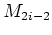. In addition we need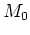for the relaxation term. Furthermore relaxation times and mobilities depend on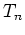, which needs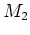(and) for its calculation.

Equation 2.74 gives an infinite hierarchy of equations. Ifis fixed, we get the self-consistent electrical field from the Poisson equation. Then we can calculateby solving the linear Equation 2.74 (with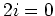) for. For orderthis is a nonlinear equation due to the dependence of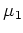onandvia the temperature.

In an infinite chain we then can solve linear equations with linear operator 2.76 to get,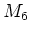, and so on. The linearity is due to the exclusive dependence of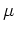and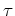onandvia the temperature. We also see that the infinite hierarchy has a solution for arbitrary chosen. This is a weakness of the model, the infinite hierarchy represents an underdetermined system of equations and is not equivalent to the full Boltzmann equation. The infinite system lacks a closure condition or constraint which picks out the one'' solution. In Section 3.2 we propose to apply the maximum entropy principle for this purpose.

As the infinite hierarchy is not equivalent to the Boltzmann equation it is reasonable to truncate the series at a low order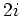. We then have to impose an additional equation for the highest occurring moment with order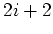, to get an equal number of equations and unknowns. This closure condition is the topic of the next chapter.